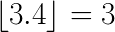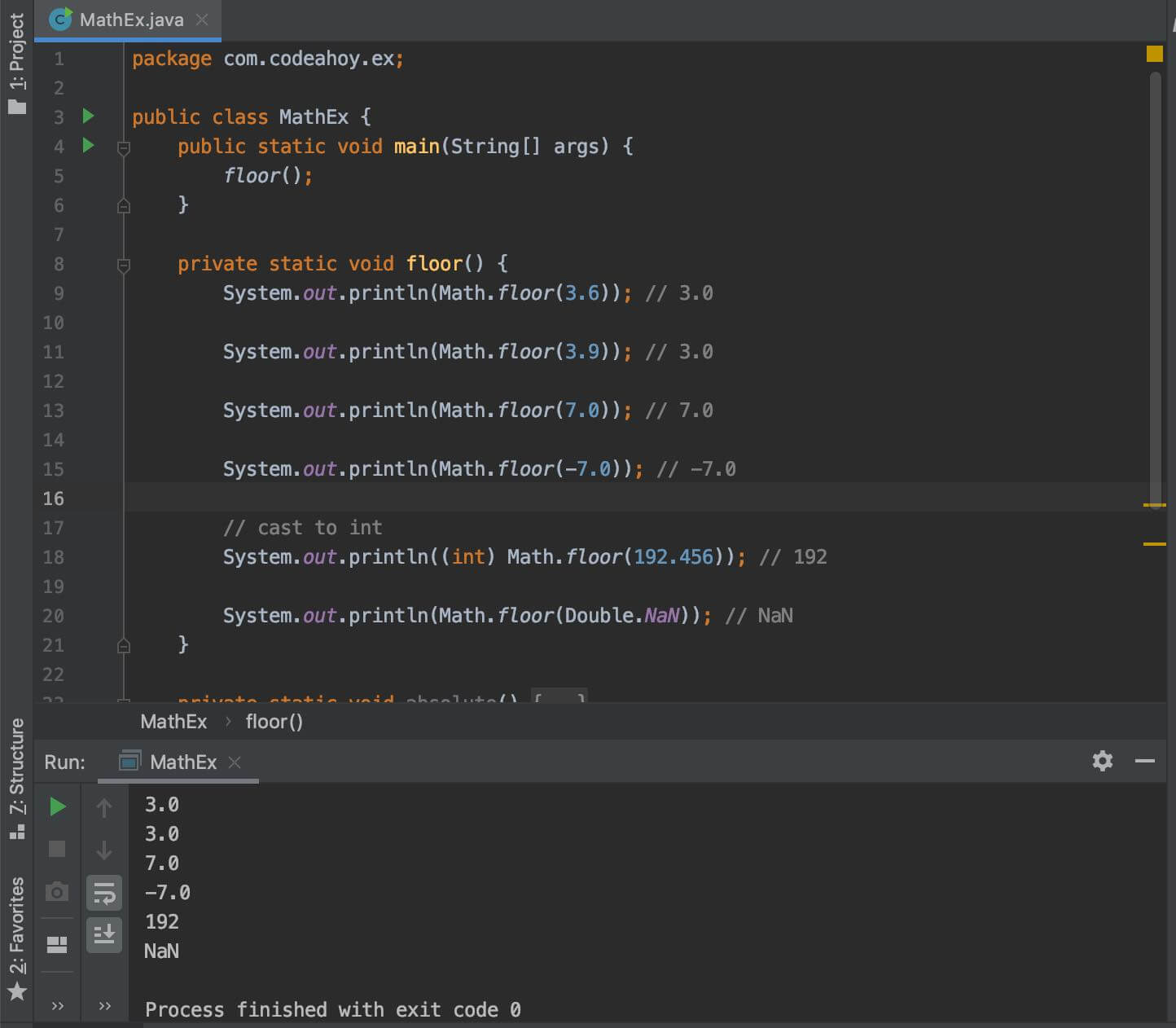# Java Math.floor() Method with Examples

Oct 12, 2019 · 2 mins readThe java.lang.Math class that comes bundled with Java contains various methods for performing basic numeric operations such as the elementary exponential, logarithm, square root, and trigonometric functions.

Math.floor(double) method is part of the Math class. We use it to find the floor value of a number. This function returns the largest integer less than or equal to argument provided. In other words, it takes a decimal and squishes it downs to its nearest integer. For example, if you pass it 3.4, it will return 3.Let’s look at its method signature:

``````public static double floor(double a)
``````

It takes an argument of type `double` and returns a value as a `double` that is less than or equal to the argument and is equal to a mathematical integer.

``````System.out.println(Math.floor(3.7)); // 3.0
``````

## Code Example

The following example illustrates how to use Math.floor(double) method.

Output

``````3.0
3.0
7.0
-7.0
192
NaN
``````

Here’s a screenshoot of the code above if you’re on mobile and having trouble reading the code above.### Special cases

• If the argument value is already equal to a mathematical integer, then the result is the same as the argument.
• If the argument is NaN or an infinity or positive zero or negative zero, then the result is the same as the argument.

If you need more detailed information, please see Javadocs. It’s worth noting that the `Math` class in Java contains several other useful methods for arithmetic, logarithms and trignometric operations.

#### You May Also Enjoy

If you like this post, please share using the buttons above. It will help CodeAhoy grow and add new content. Thank you!